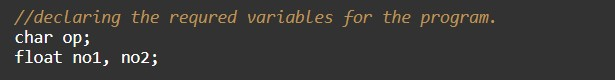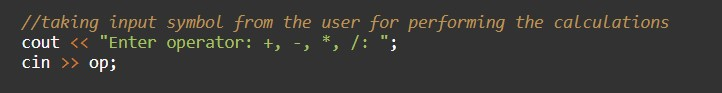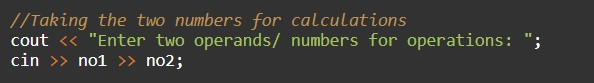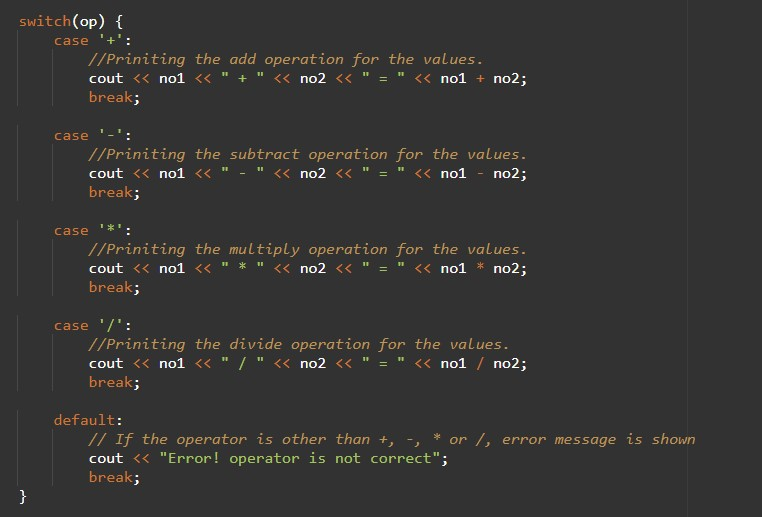# C++ Program to perform addition, subtraction, multiplication and division using Switch

In this tutorial you will learn about the C++ Program to perform addition, subtraction, multiplication and division using Switch and its application with practical example.

## C++ Program to Perform Addition, Subtraction, Multiplication, and Division using Switch

In this tutorial, we will learn to create a C++ program. After that will perform Addition, Subtraction, Multiplication, and Division in C++ programming.

## Prerequisites

Before starting with this tutorial we assume that you are best aware of the following C++ programming topics:

• Operators in C++ Programming.
• Basic Input and Output function in C++ Programming.
• Basic C++ Programming.

## Program for performing the Addition, Subtraction, Multiplication, and Division:-

Every Programming language is having many predefined functions for arithmetic operations. In the C++ programming language, we will do the Addition, Subtraction, Multiplication, and Division operations with the help of Arithmetic operators using the switch case.

## Output:-

In the above program, we have first initialized the required variable.• no1= it will hold the input number value from the user.
• no2 = it will hold the input number value from the user.
• op = it will hold the character value for the operator.

Taking Input operator from the user.Taking input number from the user.Program code for operations.In this tutorial we have learn about the C++ Program to perform addition, subtraction, multiplication and division using Switch and its application with practical example. I hope you will like this tutorial.